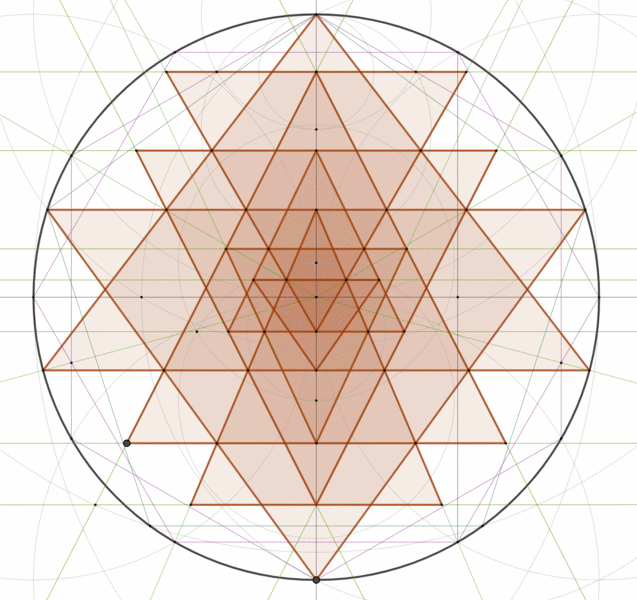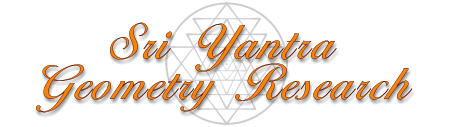Is Perfect Precision Possible? I have mentioned in a previous article that perfect precision (concurrency at the triple intersections) was not possible when drawing the Sri Yantra by hand or even when using a computer. The reasoning behind this statement comes from the method that has been used to solve the geometry mathematically. The incremental approach that has been devised so far is achieved by iterated approximation as shown in the paper from C.S. Rao. This approach allows one to get very close to perfection but never quite reaches it in a mathematical sense. The errors can be made infinitely small, but they still exist. There are a multitude of methods to draw the figure by hand, but none has ever achieved perfect precision either. Here again we are talking about mathematical precision, not visual precision. It is certainly possible to make a hand-drawn figure where the errors are smaller than the thickness of the lines used in the drawing. This has the effect of hiding the concurrency errors. However if the drawing was scaled up, the errors would eventually show up as can be seen in the animation below. The best tool to test the precision of hand-drawn methods is Geogebra, a simple and free drawing software that allows to draw geometries in a very exact way. Geogebra is different from most other drawing softwares in that it uses equations to represent points, lines, circles, etc. This makes it possible to draw geometric figures with perfect mathematical precision. Alessandro Chiodo, a mathematician from the Institute of Mathematics of Jussieu in Paris, appears to have succeeded in finding a method to hand-draw the Sri Yantra without any errors by using the Apollonius solution to the circle-line-point problem. Problem of Apollonius explained here and an interactive demonstration here. This is a major achievement. This has never been done before as far as I know. The paper by Chiodo details the method used to produce a perfectly concurrent Sri Yantra by hand (rule and compass method). Note that the first section of the paper is in French (summary) but the rest is in English. I have to warn the reader that the method is fairly complex and requires a great deal of patience and attention to details to be completed successfully. An easier way is to use the interactive Geogebra drawing at the end of this article. Using this method I was able to generate a vector Sri Yantra with perfect concurrency. The animation below from Chiodo shows the entire drawing method: Click on the image below to see his interactive Geogebra drawing of the Sri Yantra.Is Perfect Precision Possible? I have mentioned in a previous article that perfect precision (concurrency at the triple intersections) was not possible when drawing the Sri Yantra by hand or even when using a computer. The reasoning behind this statement comes from the method that has been used to solve the geometry mathematically. The incremental approach that has been devised so far is achieved by iterated approximation as shown in the paper from C.S. Rao. This approach allows one to get very close to perfection but never quite reaches it in a mathematical sense. The errors can be made infinitely small, but they still exist. There are a multitude of methods to draw the figure by hand, but none has ever achieved perfect precision either. Here again we are talking about mathematical precision, not visual precision. It is certainly possible to make a hand-drawn figure where the errors are smaller than the thickness of the lines used in the drawing. This has the effect of hiding the concurrency errors. However if the drawing was scaled up, the errors would eventually show up as can be seen in the animation below. The best tool to test the precision of hand-drawn methods is Geogebra, a simple and free drawing software that allows to draw geometries in a very exact way. Geogebra is different from most other drawing softwares in that it uses equations to represent points, lines, circles, etc. This makes it possible to draw geometric figures with perfect mathematical precision. Alessandro Chiodo, a mathematician from the Institute of Mathematics of Jussieu in Paris, appears to have succeeded in finding a method to hand-draw the Sri Yantra without any errors  by using the Apollonius solution to the circle-line-point problem. Problem of Apollonius explained here and an interactive demonstration here. This is a major achievement. This has never been done before as far as I know. The paper by Chiodo details the method used to produce a perfectly concurrent Sri Yantra by hand (rule and compass method). Note that the first section of the paper is in French (summary) but the rest is in English. I have to warn the reader that the method is fairly complex and requires a great deal of patience and attention to details to be completed successfully. An easier way is to use the interactive Geogebra drawing at the end of this article. Using this method I was able to generate a vector Sri Yantra with perfect concurrency. The animation below from Chiodo shows the entire drawing method: Click on the image below to see his interactive Geogebra drawing of the Sri Yantra.